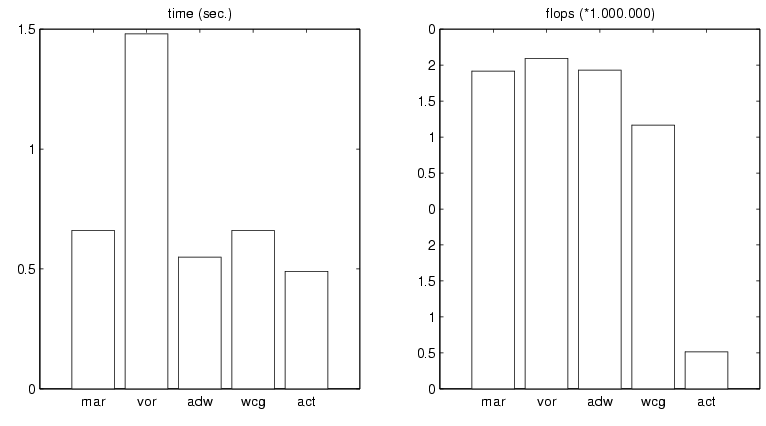### A FIRST GUIDED TOUR ON THE IRREGULAR SAMPLING PROBLEM

#### Introduction

The irregular sampling problem is concerned with the problem of recovering a band-limited signal x[n] with bandwidth M from a sequence of samples which may be taken in an irregular way.  Typically, iterative algorithms are used to recover the signal step by step from the sampling values.

Let us first describe the situation for the one-dimensional case of vectors of finite length.  Such a vector (real- or complex-valued) x[n] has spectrum in some set Omega (of its frequency domain) if all of the Fourier coefficients of x[n] which correspond to parts of the frequency domain outside Omega are zero.  Typically, Omega will be some interval around the "origin" of the frequency domain.

Fig.1(To distinguish the signal x[n] from its samples, see below, we draw it as a continuous signal. For more details about the Fast Fourier Transform and Labelling in MATLAB consult "The FFT-Guide".)

#### The Case of Regular Sampling

Before explaining the case of irregular sampling, let us recall the case of regular sampling.  If the signal length of x[n] is N, then this means that one can sample this signal at a distance a, being  kept fixed throughout the vector x[n], with a being divisor of N.  Fig.2 shows the effect of sampling of a signal of length N=288 by a distance of a=4.  Up to normalization, the Fourier transform y_s[k] of the sampled signal x_s[n] coincides with the 4-periodized version of the original sampling sequence x[n].

Fig.2This indicates that complete reconstruction of the signal x[n] from such a periodized spectrum should be easily possible as long as the shifted copies of the spectrum y[k] do not overlap.  The periodization constant being exactly N/a for a given sampling rate a, it turns out that the sampling rate should satisfy the so-called Nyquist criterion a<=N/M.  For fixed Omega, any band-limited signal with spectrum in Omega can be completely reconstructed from regular samples, as long as the shifted versions of Omega (in the cyclic sense, by multiples of N/a) do not overlap with Omega.   Indeed, in this case one simply has to multiply y_s[k] by some sequence h, with h(omega) == 1 for omega in Omega, and zero on all those shifted copies of Omega.  Explicitly, if Omega is an interval of length M=30, then any divisor a of N will do which satisfies aM <= N, see also Fig.3:

Fig.3Since multiplication on the frequency side corresponds to convolution on the time-side, it is of interest also to have a look at the ''window'' g whose Fourier transform equals a given sequence, h=fft(g), for different choices of h.  Fig.4 gives three examples of such windows, for the case N = 288 and M=30.

Fig.4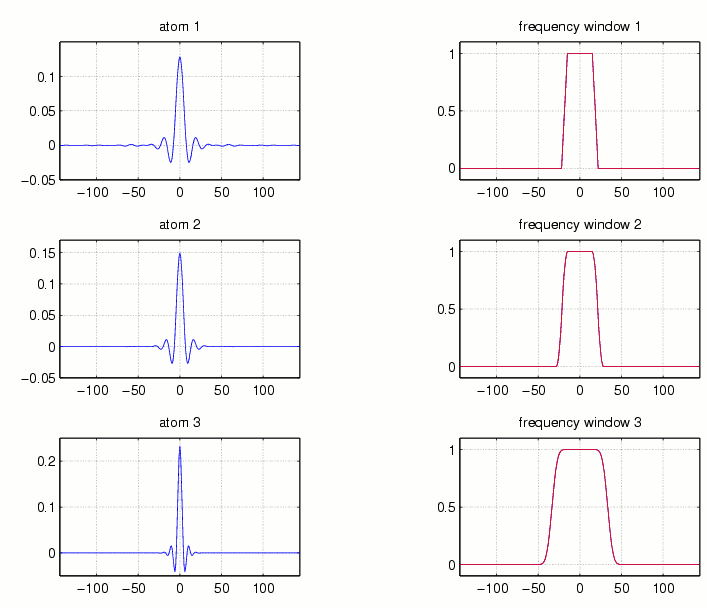For the case of critical sampling (aM = N), cf. Fig.2, the shifted copies of Omega are sitting side by side and there is no other choice for h than the indicator function of Omega.  In this case g is just (a discrete variant) of the well-known SINC function, which, however, is known to have fairly poor decay properties.  In contrast, for the case of ''oversampling'' (where one has more samples than absolutely necessary), i.e. a is smaller than N/M, N/a will be large compared to Omega.  This gives some freedom in the choice of h (instead of an indicator function one can e.g. choose a trapezoid like function with decent decay at the ends).  The corresponding window g of a smooth function h will be better concentrated near the origin.
Since convolution of the sampled signal with the window g can be reinterpreted as forming a series of shifted copies of g, with the given sampling coordinates being the coefficients of the series expansion (linear combination), the better decay of g will result in better locality properties of the reconstruction algorithm.  In other words, it is not necessary to have all the samples available in order to reconstruct a part of a signal.  The use of well localized ''atoms'' g implies that the following (very natural) principle applies, indicating that the truncation error can be reasonably kept within limits: Given some interval of interest it is only necessary to use the samples in some neighborhood of the given interval in order to achieve a small reconstruction error there, see Fig.5:

Fig.5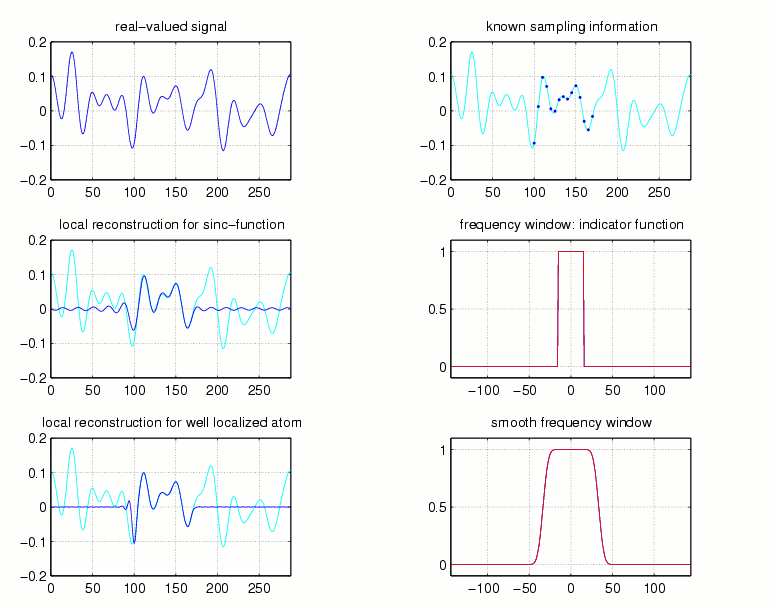#### The Case of Irregular Sampling

Let us now come to the situation of irregular sampling.  For the rest of the presentation we will use a typical real-valued band-limited signal of length N=288, and a random sampling set (the maximal gap being close to the Nyquist gap for the given spectral support Omega, which consists of M=30 frequencies in this case).  Fig.6 shows the signal x[n], its Fourier transform y[k], the irregular sampled version of x[n] (with unknown samples taken as zeroes), and the Fourier transform of the sampled signal, clearly indicating that the simple recovery method will not give complete reconstruction.  (But at least near the origin in the frequency domain there is some similarity between the original signal and its irregular sampled version.)

Fig.6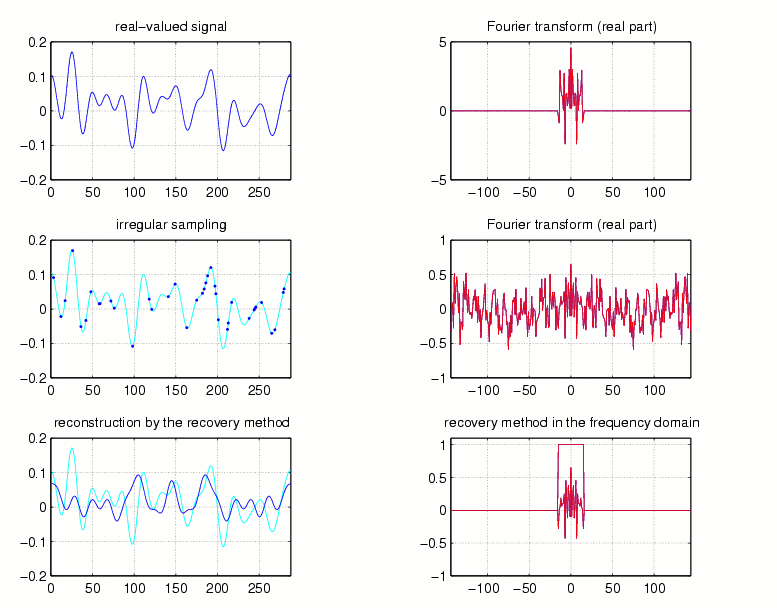Most of the irregular sampling algorithms are iterative in nature.  Starting from some initial guess, typically based on the given sampling values, further approximations are obtained step by step, using the available (assumed) knowledge about the size and location of Omega (in some cases an educated guess concerning Omega has to be made).  As a first example, let us explain the so-called Voronoi-method.  Here we start with nearest neighborhood interpolation.  In other words, given the sampling values at known positions we form a step function which is constant from midpoint to midpoint of the given sampling sequence.  In the case where the number of missing points between subsequent sampling positions is odd, the midpoint itself is assigned the arithmetic mean of the two adjacent sampling points.  Because of the assumed smoothness of the signal, this step function will not be too far away from the original signal, in the mean-squared sense.  Having used the sampling values, the information concerning Omega can be used next.  The step function (which appearently contains many high frequencies outside of Omega) is filtered, i.e. only those Fourier coefficients which belong to Omega are preservered, the rest is discarded.  This operation corresponds to a simple projection operator.
Fig.7 shows this step-function, the original sampling points plotted as black points, and its Fourier transform in the first line.  Below, the filtered version is displayed.  It is the first step of the iterative Voronoi approach (or Allebach-algorithm).

Fig.7Clearly the filtering process destroys the pointwise interpolation property of the Voronoi step-function, but theoretical considerations show that (given Omega and the sampling rate in terms of the maximal gap) one can give the following guarantee:  If the maximal gap is smaller than the Nyquist rate (N/M), then the following iterative scheme will converge to the original signal x[n] at a geometric rate (which will be the better the smaller the actual maximal gap is compared to the Nyquist rate).
Indeed, it can be shown that there is some factor q < 1, such that the Omega-filtered version x_1[n] of the nearest neighborhood interpolation satisfies ||x-x_1|| < q ||x||.  We therefore look at the difference signal x[n]-x_1[n].  According to the construction, x_1[n] has its spectrum within the same set Omega, and for obvious reasons we know its coordinates at the given sampling positions.  Therefore, the estimate indicated above can be applied and we can again recover a certain portion of the remaining signal by repeating the first step, now starting with the sampled coordinates of x[n]-x_1[n].  Technically speaking we recover 100(1-q) percent of the missing energy of the difference signal x[n]-x_1[n] at the second step.  Continuing to use the difference between the given sampling values of x[n] and those of the n-th approximation we generate additive corrections which lead stepwise to improved approximations.

Fig.8 shows the approximations of the Allebach-algorithm and their errors after 1, 5, and 20 iterations.

Fig.8To give an idea of the rate of convergence depending on the maximal gap of the sampling set, Fig.9 compares four sampling sets by using the Voronoi-Allebach algorithm (set2 corresponds to the sampling set used in Fig.8).

Fig.9The Voronoi-approach is just one method to get a bandlimited approximation of the original signal from its sampling points.  As one can guess, there exist some more.  Indeed, if we use either a trivial interpolation (where all the unknown points are set equal to zero) or the so-called adaptive-weights-approximation (where every sample point is multiplied with a weight-factor depending on its distance to its neighbor sampling points), and if we proceed as in the Allebach-algorithm, then we get to other reconstruction methods known as the Marvasti-algorithm  and the adaptive-weights-algorithm, respectively.  To prove their convergence one can apply the same arguments as for the Voronoi-Allebach-algorithm.

Fig.10 and Fig.11 show the approximations and the corresponding errors of the two algorithms after 1, 5, and 20 iterations.

Fig.10: Marvasti-algorithm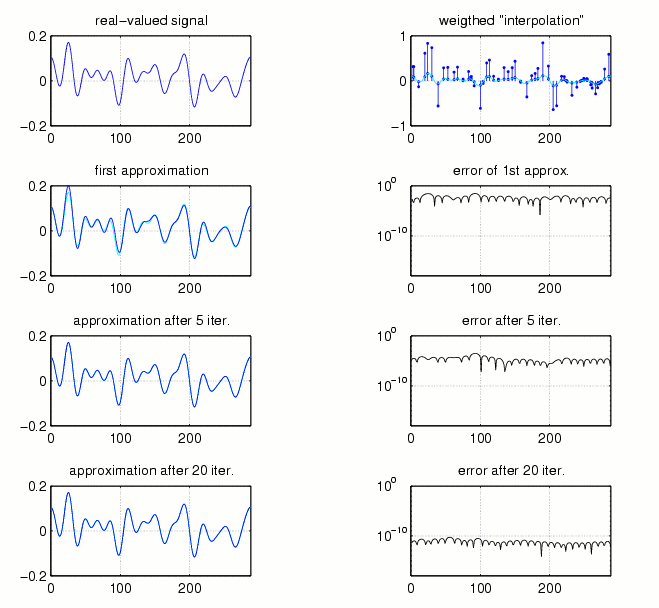In order to visualize the difference between those algorithms, Fig.12 compares rates of convergence in terms of the reconstruction error, time, and number of floating point operations used for 20 iterations of the Voronoi-Allebach-algorithm (vor), the Marvasti-algorithm (mar), and the adaptive-weights-algorithm (adw) for the sampling sequence used in Fig.8, Fig.10, and Fig.11 .

Fig.12The adaptive-weights-method can be improved by using the conjugate gradient algorithm (cg), a very efficient method to solve iteratively a positive definite, symmetric, linear system.  In fact, the first step of the adw-algorithm consisting of discarding high frequencies of the "weighted interpolation" of the signal x[n] in order to get the first approximatio x_1[n], can be replaced by the matrix- multiplication Sx=x_1, where S is of the form S=CC'.  Let P be the number of irregular samples, then C is an (N,P)-matrix whose columns are the shifted (by the sampling points) versions of an appropriate atom on the time-side,  C' denotes the transpose of C.  It can be shown that S is positive definite and symmetric (Frame-theory). Applying the cg-method to Sx=x_1 in order to approximate the signal x[n] leads to the adaptive-weights-conjugate-gradient-algorithm (wcg).  Note that S is completely determined by the known sampling information.

Fig.13 compares the wcg-algorithm with the Voronoi-method and the adaptive-weights-algorithm.

Fig.13:In the discrete case a band-limited signal can be written as a trigonometric polynomial.  Using the Dirichlet kernel as atom on the time-side and introducing trigonometric polynomials, the linear system Sx=x_1 can be transformed into a system of a positive definite Toeplitz matrix, a matrix whose entries are constant along each diagonal.  For the solution of such Toeplitz systems the cg-algorithm is an even more powerful acceleration method.  The combination of adaptive weights, conjugate gradient, and Toeplitz systems forms the adaptive-conjugate-Toeplitz-algorithm (act), which is a very efficient iterative algorithm to reconstruct a band-limited signal from irregular sampling values.

The comparison between the adw-method, the wcg-method, and the act-algorithm is shown in Fig.14 .

Fig.14:As a last example we compare all the algorithms mentioned above by taking a sampling sequence whose maximal gap is three times larger than the Nyquist gap, such that no theoretical guarantee for convergence can be given.  In case of convergence the behavior of the error in terms of iterations can be considered as a mesure of stability.

Fig.15:Fig.16: